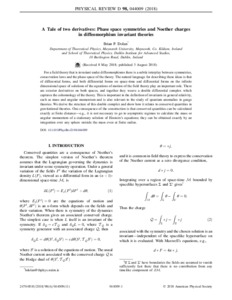# A Tale of two derivatives: Phase space symmetries and Noether charges in diffeomorphism invariant theories

Dolan, Brian P. (2018) A Tale of two derivatives: Phase space symmetries and Noether charges in diffeomorphism invariant theories. Physical Review D, 98 (044009). ISSN 1550-7998Previewmore...Add this article to your Mendeley library

## Abstract

For a field theory that is invariant under diffeomorphisms there is a subtle interplay between symmetries, conservation laws and the phase space of the theory. The natural language for describing these ideas is that of differential forms, and both differential forms on space-time and differential forms on the infinite dimensional space of solutions of the equations of motion of the field theory play an important role. There are exterior derivatives on both spaces, and together they weave a double differential complex which captures the cohomology of the theory. This is important in the definition of invariants in general relativity, such as mass and angular momentum and is also relevant to the study of quantum anomalies in gauge theories. We derive the structure of this double complex and show how it relates to conserved quantities in gravitational theories. One consequence of the construction is that conserved quantities can be calculated exactly at finite distance—e.g., it is not necessary to go to asymptotic regimes to calculate the mass or angular momentum of a stationary solution of Einstein’s equations; they can be obtained exactly by an integration over any sphere outside the mass even at finite radius.

Item Type: Article The author acknowledges support by the Action MP1405 QSPACE from the European Cooperation in Science and Technology (COST). Phase space symmetries; Noether charges; diffeomorphism invariant theories; Faculty of Science and Engineering > Mathematical Physics 10480 https://doi.org/10.1103/PhysRevD.98.044009 Dr. Brian Dolan 13 Feb 2019 16:20 Physical Review D American Physical Society Yes European Cooperation in Science and Technology (COST)Item control page